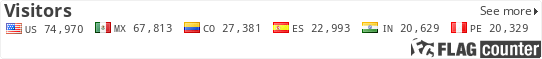### Total Factor Productivity

Total Factor Productivity is a measure of the physical output produced from the use of a given quantity of inputs by the firm. When having multiple outputs and multiple inputs, the ratio of the weighted sum of outputs with respect to the weighted sum of inputs is used to calculate the Total Factor Productivity Index. In general, the weights are the cost share for inputs and the revenue shares for the outputs.

Prices or cost shares and revenue shares may change between two periods. There are two alternatives in dealing with this problem which implies different calculations: same weights may be used in both periods, or each period may use a different weight. If the price index does not have the same scope (e.g., the input price index is related to the economy in general and the output price index is related to the particular industry), the TFP index will be biased.

A final note should be made about measuring the TFP index change for a group of firms: pair wise comparisons will not necessarily give the same ranking of firms. These indexes need to be adjusted using a more complex calculation technique.

• Advantages of Index Methods: The approach only requires data on two observations, such as two firms or two time periods.
• Disadvantages of Index Methods: TFP cannot be decomposed into the different types of efficiencies (i.e. technical, allocative and economic, as mentioned earlier).
• Application of Index methods: An example of an analysis using the TFP index method in the water and sanitation sector is an assessment of the performance after privatization in England and Wales